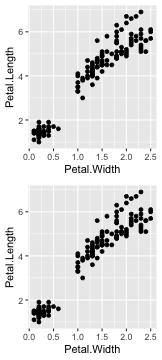This document explains concepts and basics of {ggfortify}. {ggfortify} helps plotting some popular R packages with {ggplot2} in a unified way. See github to check the list of supported packages / classes.

library(ggfortify)

Using ggplot2::autoplot

This is the easiest way provided by {ggfortify}. Calling autoplot with supported instance should output appropriate plot based on its type, as standard plot function does.

autoplot(AirPassengers)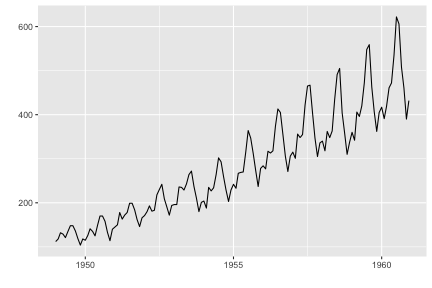You can specify some options to control how plot looks. As is often the case with statistic classes, there are some objects to be drawn, such as actual value, predicted value and confidence interval, etc. Thus, each plotting option has a dot-separated format like <target name>.<ggplot option name> such as ts.colour and conf.int.linetype.

These options can be used for the similar type of instances commonly. For example, ts.colour works for all time-series-likes (there is no separate options like xts.colour and timeSeries.colour, etc). To check available options, use help(autoplot.ts) or help(autoplot.*) for any other objects.

autoplot(AirPassengers, ts.colour = 'blue')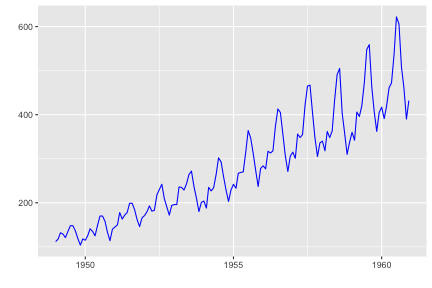Decorating Plots

autoplot returns ggplot instance. Thus, you can decorate as the same as in {ggplot2}. To make autoplot API simple, {ggfortify} only offers some limited options which must be done during ggplot instance creation. Additional decoration should be done against the returned ggplot instance.

p <- autoplot(AirPassengers)
class(p)
##  "gg"     "ggplot"
# plot as it is
pp + ggtitle('AirPassengers') + xlab('Year') + ylab('Passengers')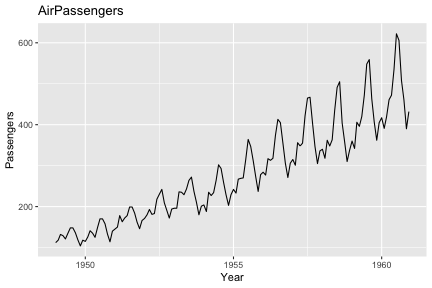# these common options are supported as keywords
autoplot(AirPassengers, title = 'AirPassengers', xlab = 'Year', ylab = 'Passengers')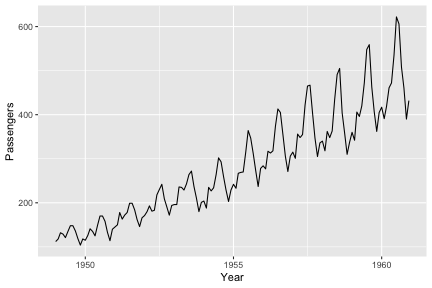set.seed(1)
p <- autoplot(kmeans(iris[-5], 3), data = iris)
# plot as it is
p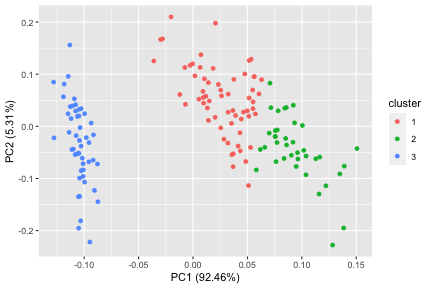# change colour mapping
p + scale_colour_brewer()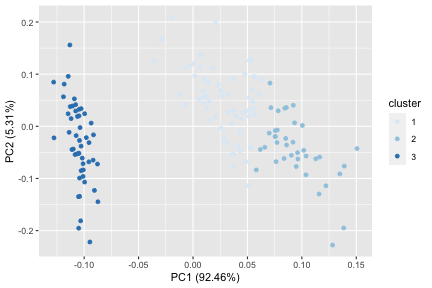Want Different Plots?

Internally, autoplot calls a generic function named ggplot2::fortify to convert the passed instance to data.frame. {ggfortify} defines fortify function for all supported classes. If you want a different type of plot, you can use fortify to get data.frame, then call ggplot in a normal way.

Following example shows a bar plot counting records per k-means clusters.

df <- fortify(kmeans(iris[-5], 3), data = iris)
##   Sepal.Length Sepal.Width Petal.Length Petal.Width Species cluster
## 1          5.1         3.5          1.4         0.2  setosa       1
## 2          4.9         3.0          1.4         0.2  setosa       1
## 3          4.7         3.2          1.3         0.2  setosa       1
## 4          4.6         3.1          1.5         0.2  setosa       1
## 5          5.0         3.6          1.4         0.2  setosa       1
## 6          5.4         3.9          1.7         0.4  setosa       1
ggplot(df, aes(x= cluster, fill = cluster)) + geom_bar()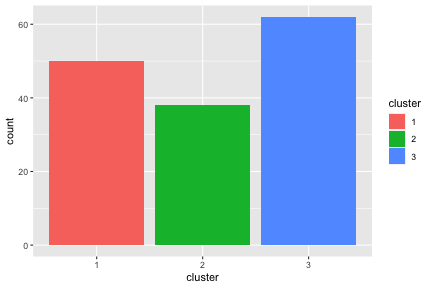Handling Subplots

autoplot may draw subplots in some classes. For exmple, autoplot.lm draws diagnostics plots as below.

res <- lm(Volume ~ Girth, data = trees)
mp <- autoplot(res, ncol = 4)
mp

Because {ggplot2} itself cannot handle different kinds of plots in a single instance, {ggfortify} handle them using its original class named ggmultiplot. You can use + operator to decorate ggmultiplot.

class(mp)
mp + theme_bw()

Also, + operator appends plots if ggplot or ggmultiplot instance is given as right-hand-side. Following example attaches 2 scatter plots after diagnostics.

mp +
(ggplot(trees, aes(Girth, Volume)) + geom_point()) +
(ggplot(trees, aes(Girth, Height)) + geom_point())

You can extract subset using [ and [[ operators.

mp[2:3]
mp[]

You can extract arbitrary subset, modify, and draw.

mp[2:3] <- mp[2:3] + theme_bw()
mp

Draw Multiple Instances as Subplots

Assuming you want to plot multiple instances at once. {ggfortify} can accept list of supported instances and can output subplots.

Following example shows 3 kmeans plots changing number of clusters.

res <- lapply(c(3, 4, 5), function(x) kmeans(iris[-5], x))
autoplot(res, data = iris[-5], ncol = 3)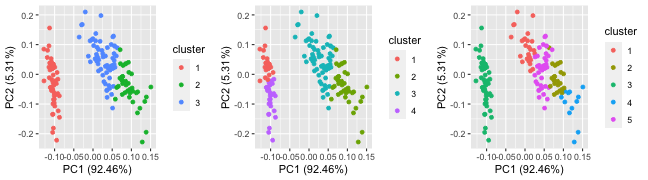Next example shows multiple survival curves as subplots.

library(survival)
res <- list(a = survfit(Surv(time, status) ~ 1, data = lung),
b = survfit(Surv(time, status) ~ sex, data = lung))
autoplot(res)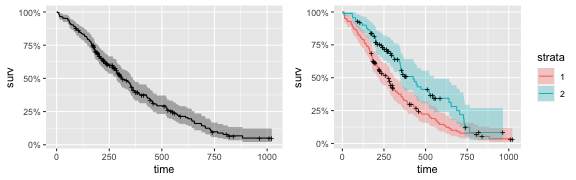You can pass a list contains different classes to autoplot.

library(vars)
res <- list(a = Canada, b = AirPassengers)
autoplot(res)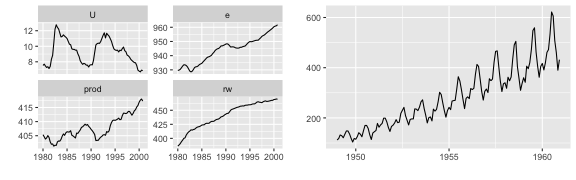You can instanciate ggmultiplot from a list of ggplot instances.

p1 <- ggplot(iris, aes(Petal.Width, Petal.Length)) + geom_point()
p2 <- ggplot(iris, aes(Petal.Width, Petal.Length)) + geom_point()
new('ggmultiplot', plots = list(p1, p2))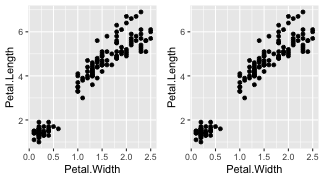Layout can be specified via nrow and ncol keywords.

new('ggmultiplot', plots = list(p1, p2), ncol = 1)